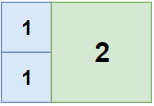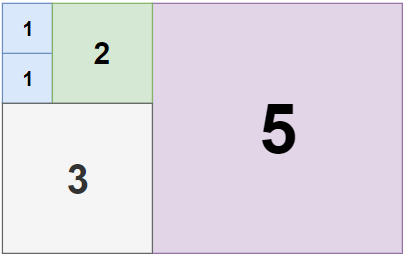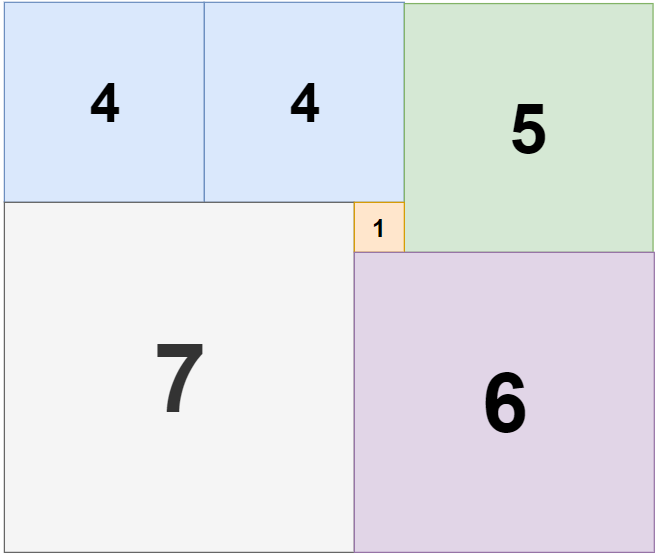# GeetCode Hub

Given a rectangle of size `n` x `m`, find the minimum number of integer-sided squares that tile the rectangle.

Example 1:```Input: n = 2, m = 3
Output: 3
Explanation: `3` squares are necessary to cover the rectangle.
`2` (squares of `1x1`)
`1` (square of `2x2`)```

Example 2:```Input: n = 5, m = 8
Output: 5
```

Example 3:```Input: n = 11, m = 13
Output: 6
```

Constraints:

• `1 <= n <= 13`
• `1 <= m <= 13`

class Solution { public int tilingRectangle(int n, int m) { } }